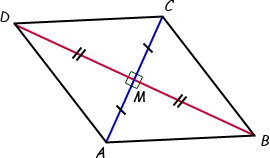SEARCH HOMEMath Central Quandaries & QueriesQuestion from Jeanie, a student: How do you prove that a quadrilateral is a rhombus because the diagonals of the quadrilateral are perpendicular and bisect each other using the 2-column proof method?Hi Jeanie. Start with a diagram, labelling the corners, intersection point and marking what is perpendicular and how the bisections make sides equally long:Can you see four right triangles? Now you can see that all four have the same Side-Angle-Side (S.A.S.) so all four triangles are congruent. What does this tell you about the lengths of the four hypotenuses that form the sides of quadrilateral ABCD? What do you have to show to prove the quadrilateral is a rhombus?

The two-column method is simply a way of keeping your reasoning logical. In the left column, you write your statements which are either givens or logically deduced from statements above. In the right column, you write your reasons why the statement on the left is valid. Here's an example.

Cheers,
Stephen La Rocque.Math Central is supported by the University of Regina and The Pacific Institute for the Mathematical Sciences.